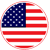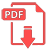# Home | Mathematics | Pre-Algebra

## Equations with fractions

When solving a simple equation, think of the equation as a balance, with the equals sign (=) being the fulcrum or center. Thus, if you do something to one side of the equation, you must do the same thing to the other side. Doing the same thing to both sides of the equation (say, adding 3 to each side) keeps the equation balanced.English